# Ten things you (possibly) didn’t know about the Petersen graph

Some mathematical objects are completely boring. Others have a few interesting properties. The Petersen graph, by comparison, has a plethora of quite remarkable features that, taken alone, would each qualify the graph as being interesting. I’ve mentioned this graph a few times before, but this is the first post exclusively dedicated to it. Firstly, here’s a picture of the graph: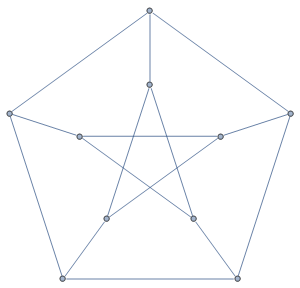For completeness, I’ll mention that it’s a 3-regular graph with 10 vertices and 15 edges, and that it is both vertex- and edge-transitive. Now, let’s discuss why it’s interesting:

Wagner’s theorem is well known, and states that a graph is non-planar if and only if it contains either the complete graph K5 or the bipartite graph K3,3 as a graph minor. The Petersen graph has both of these as minors, so it is really non-planar! It has the stricter property that if you draw the Petersen graph in three-dimensional space (with no edges crossing), you can necessarily find two linked cycles.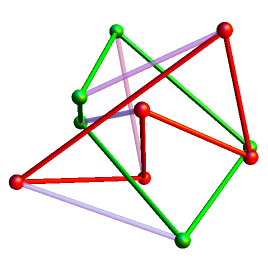In fact, like planar graphs, the linklessly embeddable graphs are closed under the operation of taking graph minors. The Petersen graph, together with K6 and five other graphs, form a finite set of forbidden minors (the existence of which is guaranteed by the Robertson-Seymour theorem together with Zorn’s lemma). They all have 15 edges, and can be interconverted by delta-wye transformations.

### 2. Toroidal and projective-plane embeddings

In the previous section, I mentioned how the Petersen graph is linklessly embeddable, therefore far from being planar. Consequently, removing any vertex of the Petersen graph leaves a non-planar graph. Hence, it is somewhat surprising that it can be drawn on the surface of a torus without any edges crossing. Indeed, it can be shown that every generalised Petersen graph (formed by taking the skeleton of a regular n-gonal prism and replacing one of the terminal n-gons with a regular star n-gon) is toroidal.

Edit: The Desargues graph is not toroidal, so the previous statement is erroneous. Thanks go to Taylor Ball for noticing this fallacy!

The Petersen graph has a more natural and very symmetric embedding on the projective plane. It can be obtained by drawing a regular dodecahedron on the surface of a sphere, and identifying antipodal points, edges and faces. This results in an abstract polyhedron called the hemi-dodecahedron, the skeleton of which is the Petersen graph. The hemi-dodecahedron and hemi-icosahedron can then be used to construct even more pathological polytopes, the 11-cell and the 57-cell.

### 3. Construction as a Kneser graph

The hemi-dodecahedron has 60 symmetries, abstractly isomorphic to the alternating group A5. It follows that the automorphism group of the Petersen graph must contain A5 as a subgroup. You may intuitively think that it is precisely A5, but the subtle operation of ignoring the faces of the hemi-dodecahedron could result in additional automorphisms.

It transpires that this is indeed the case, and the Petersen graph has 120 symmetries, abstractly isomorphic to S5. The easiest way to see this is to construct the Petersen graph as a Kneser graph. Specifically, each vertex is associated with a subset of {a,b,c,d,e} of cardinality 2, and two vertices are joined with an edge if the corresponding subsets are disjoint.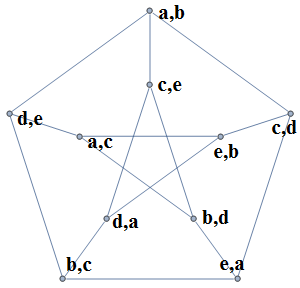To show that the only symmetries are the permutations of {a,b,c,d,e}, it suffices to demonstrate that we can recover the elements from the graph. We describe a pair of edges x, y as maximally distant if no vertex of x is connected to any vertex of y. It can easily be seen that if we select an edge x, we can find precisely two edges y, z maximally distant from x. Also, y and z are maximally distant from each other.

Hence, the 15 edges of the Petersen graph uniquely and naturally partition into 5 subsets of three edges. These are bijected with the elements of {a,b,c,d,e}, where the six vertices belonging to the union of the three edges are those not containing that element. This completes the proof that the symmetries of the Petersen graph are exactly the permutations in S5.

### 4. Not a Cayley graph

I’ve mentioned that it is vertex-transitive, that is to say that there are symmetries taking any one vertex to any other (or, equivalently, that every vertex is ‘essentially the same’). Graphs with this property are ten-a-penny, such as the following: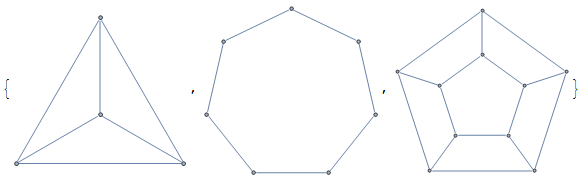These are Cayley graphs of the groups C4, C7 and D10, respectively (N.B. a group can have multiple Cayley graphs, depending on which generators are used). Every Cayley graph is vertex-transitive (by construction), but not all vertex-transitive graphs are Cayley. The smallest counter-example is, unsurprisingly, the Petersen graph.

### 5. Hypohamiltonian

A graph is described as Hamiltonian if we can find a cyclic tour, which visits every vertex exactly once. Most vertex-transitive connected graphs are Hamiltonian, and it is conjectured that there are only five counter-examples. The smallest of these is really trivial, being the complete graph K2 (once the edge is traversed, you cannot return). The others are the Petersen graph, the Coxeter graph, and two larger graphs derived from the Petersen and Coxeter graphs.

However, removing any vertex leaves a Hamiltonian graph, so the Petersen graph is described as hypohamiltonian. We can see that this is the case by using vertex-transitivity (hence only one vertex needs to be considered) and observing the following drawing of the Petersen graph: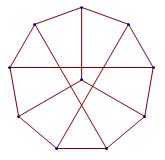Indeed, the Petersen graph is the smallest hypohamiltonian graph.

### 6. Snarks

In an earlier post, I mentioned how the four-colour theorem is equivalent to showing that no snark is planar. A snark, by the way, is a 3-regular connected graph with no isthmus* (edge whose removal would result in a disconnected graph), such that we cannot 3-colour the edges such that each vertex is incident with one edge of each colour. The dodecahedral graph, for example, is 3-regular, connected and bridgeless**, but not a snark as it admits the following edge tricolouring:The Trinity mathematician William Tutte, whom you may know from such things as the cryptanalysis of the Lorenz cipher in the Second World War and the discovery of the first perfect squared square, conjectured that every snark has the Petersen graph (the smallest snark) as a graph minor. This was proved recently, with the four-colour theorem following as a corollary.

* Some people use the alternative term ‘bridge‘. I am not one of those people…

** …but nevertheless, I do admit that ‘isthmusless’ sounds clumsy.

### 7. Desargues’ theorem and right-angled hexagon theorem

Even more recently, I wrote about Pappus’ theorem and Desargues’ theorem. The latter can be interpreted as a statement about the Desargues graph (bipartite double cover of the Petersen graph), or equivalently about the Petersen graph itself. Another theorem involving the Petersen graph is this one, mentioned in an e-mail from John Conway:

Suppose we have 10 lines in Euclidean 3-space, corresponding to the vertices of the Petersen graph. To each edge, we assign the statement ‘the lines corresponding to the two endpoints intersect at right angles’. If fourteen of the statements are true, then so is the fifteenth (thereby constituting a rather impressive example of (15,14)-TFAE).

Anyway, I suppose I ought to explain what a bipartite double cover is. Basically, we replace each vertex of the Petersen graph with a red vertex and a blue vertex, joining two vertices in the resulting graph if the vertices are different colours and the parent vertices were connected by an edge. What results is the 30-edge 20-vertex Desargues graph: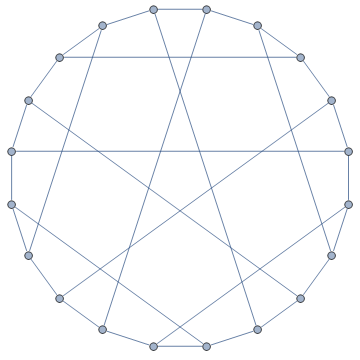This is a different from the dodecahedral graph, which can also be regarded as a double cover of the Petersen graph.

### 8. The Hoffman-Singleton theorem

The Petersen graph has diameter 2 and girth 5. In other words, the shortest cycle has length 5, and any two vertices are either adjacent or share a common vertex. This is equivalent to a set of constraints posed in an AMS question. Ignoring the empty graph, the only possible graphs are:

• The 5-vertex 2-regular pentagon;
• The 10-vertex 3-regular Petersen graph;
• The 50-vertex 7-regular Hoffman-Singleton graph;
• A particular 3250-vertex 57-regular graph whose existence and uniqueness are unknown.

Intriguingly, the Hoffman-Singleton theorem has reduced the problem of finding all graphs with this property to a finite case-bash, but where ‘finite’ is still prohibitively large for an exhaustive search. This is a counter-example to the common misconception that all finite problems are trivial.

### 9. Induced subgraphs

The Petersen graph appears as an induced subgraph in many larger interesting graphs. The aforementioned Hoffman-Singleton graph is one such example, as is the Clebsch graph (a 16-vertex 5-regular graph, such that the removal of any vertex and its five neighbours leaves the Petersen graph).

The Clebsch graph can be constructed by taking a tesseract and joining pairs of opposite vertices. It is interesting in that the edges of K16 can be partitioned into three copies of the Clebsch graph, resulting in a 3-colouring of K16 with no monochromatic triangle. This, combined with a trivial upper bound, establishes the Ramsey number R(3,3,3) = 17.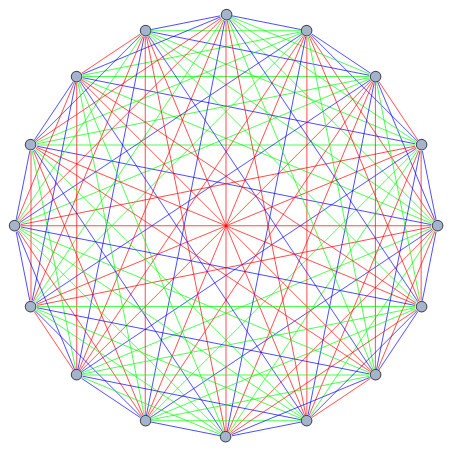Naturally, the Petersen graph appears infinitely often as an induced subgraph of the Rado graph, as noted by Gabriel Gendler in his cp4space guest post.

### 10. Cycle-continuous maps

A map from (the edges of) a graph G to a graph H is described as cycle-continuous if the pre-image of any Eulerian subgraph is also Eulerian. This definition is similar to that of continuity in a general topological space, but with Eulerian subgraphs instead of open sets. Jaeger conjectured that any bridgeless graph can be mapped cycle-continuously into the Petersen graph, and demonstrated that (if true) this would imply three more open conjectures for free:

### Conclusion

In summary, there are at least as many interesting properties of the Petersen graph as there are vertices. I shall end on a quotation in true oratory style, mentioning that Donald Knuth (inventor of LaTeX, used by mathematicians and prophylactic manufacturers alike!) described the Petersen graph as:

“a remarkable configuration that serves as a counterexample to many optimistic predictions about what might be true for graphs in general”

This entry was posted in Uncategorized. Bookmark the permalink.

### 0 Responses to Ten things you (possibly) didn’t know about the Petersen graph

1.wojowu says:

I knew about first part of 1., 5. and, thanks to your blog, 1., 4., 6. and 8. Now I understand why this graph is so awesome 🙂 Thanks to 6. it became also mine favorite graph.

2.jkiparsky says:

Just one minor quibble: Knuth invented TeX. LaTeX was developed on top of TeX by Leslie Lamport. 🙂

3.Anonymous says:

Ya,Thanks for the clarification!

4.chithra says:

is petersen graph a permutation graph?

•Yixin Cao says:

If by permutation graph you mean https://en.wikipedia.org/wiki/Permutation_graph, then the answer is no. Petersen graph has so many pentagons, hence not perfect.